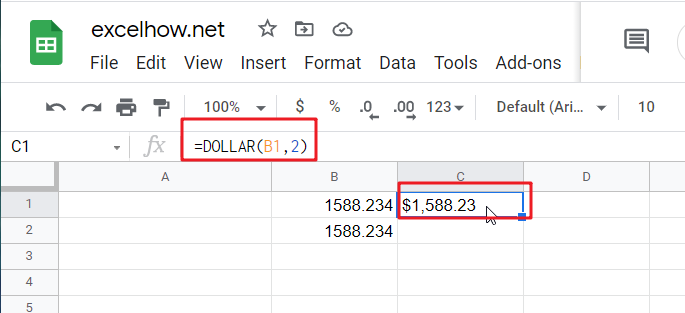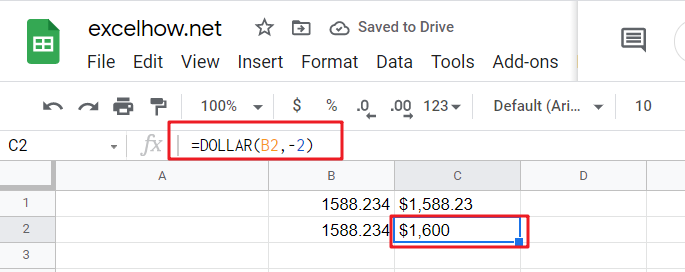This post will guide you how to use Google Sheets DOLLAR function with syntax and examples.

## Description

The Google Sheets DOLLAR function converts a number to text using currency format (such as: \$ symbol), the currency format used depends upon your default language setting on your computer.

The DOLLAR function can be used to convert a number to text using the locale-specific currency number format

The DOLLAR function is a build-in function in Google Sheets and it is categorized as a Text Function.

## Syntax

The syntax of the DOLLAR function is as below:

= DOLLAR  (number, [decimals])

Where the DOLLAR function arguments are:

• numberThis is a required argument. The number to be converted into text string. Or a reference to a cell that containing a number.
• DecimalsThis is an optional argument. It will specify the number of decimal places to be displayed after decimal point.

## Example

The below examples will show you how to use Google Sheets DOLLAR function to convert a number as text using currency format based on locale setting of your computer.

#1 To convert Number value in B1 cell to a text string of a currency format and keep 2 digits to the right of the decimal point, just using the following formula:

`=DOLLAR(B1,2)`#2 To Display the number in B2 in a currency format and keep 2 digits to the left of the decimal point, using the following DOLLAR formula in google sheets:

`=DOLLAR(B2,-2)`Notes: